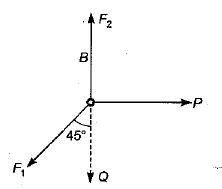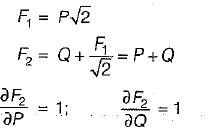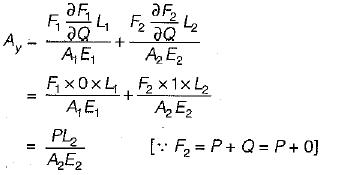Courses

# Deflection And Theories Of Failure - 2

## 10 Questions MCQ Test Topicwise Question Bank for GATE Mechanical Engineering | Deflection And Theories Of Failure - 2

Description
This mock test of Deflection And Theories Of Failure - 2 for Mechanical Engineering helps you for every Mechanical Engineering entrance exam. This contains 10 Multiple Choice Questions for Mechanical Engineering Deflection And Theories Of Failure - 2 (mcq) to study with solutions a complete question bank. The solved questions answers in this Deflection And Theories Of Failure - 2 quiz give you a good mix of easy questions and tough questions. Mechanical Engineering students definitely take this Deflection And Theories Of Failure - 2 exercise for a better result in the exam. You can find other Deflection And Theories Of Failure - 2 extra questions, long questions & short questions for Mechanical Engineering on EduRev as well by searching above.
QUESTION: 1

### The following figure shows a simply supported beam of length L which carries a concentrated load Pat B. The maximum deflection occurs at point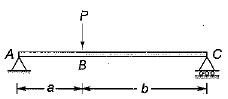Solution: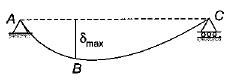QUESTION: 2

### Two equal length beams are fixed at their ends. One carries a distributed load and other carries same load but concentrated in the middle. The ratio of maximum deflections will be

Solution: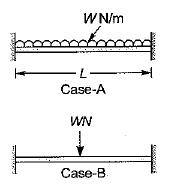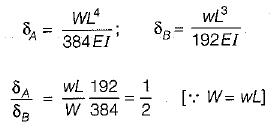QUESTION: 3

### Consider the following discontinuity functions and match it correctly, List-I (Name) with List-ll (Graph): List-I A. Unit impulse function B. Unit ramp function C. Unit step function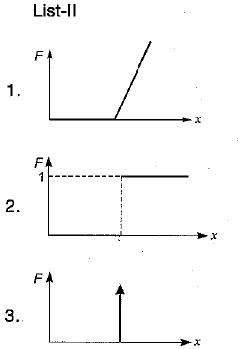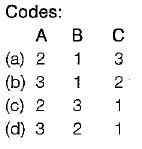Solution:
QUESTION: 4

What is deflection at A of the following cantilever beam with two different moments of inertia?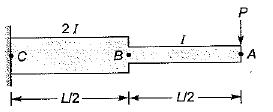Solution:

Deflection of A due to bending is AB as a cantilever beam: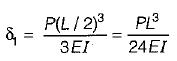Part CB also behaves as a cantilever and contributes to the deflection of point A.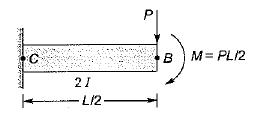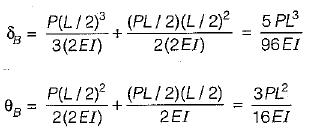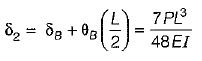Total deflection of free end,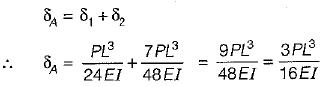The deflection is found out by method of superposition is very useful for finding deflections of nonprismatic beams.

QUESTION: 5

Which of the following statements is INCORRECT?

Solution:
QUESTION: 6

A cantilever of span 4 m carries a point load at the free end. If the slope is 2°(0.035 radian), the deflection at the free end would be nearest to

Solution: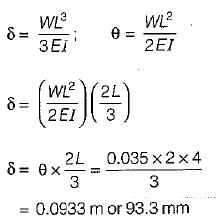QUESTION: 7

A beam 3 meters in length is simply supported at its ends and carries a point load W at its center. If the slope at the ends is not to exceed 1 °(0.01745 radian), the limiting deflection at the center of beam would be

Solution: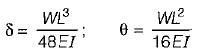∴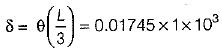= 17.45 mm

QUESTION: 8

Consider the following theorem related to strain energy: “For a linear elastic structure subjected to two sets of forces, the work done by the first set through the displacement produced by the second set is equal to the work done by the second set through the displacements produced by the first set’’ The theorem is

Solution:
QUESTION: 9

The internal strain energy stored within an elastic bar subjected to a torque T is

Solution: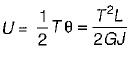QUESTION: 10

The bars AB and CB of following figure are pinned at A, C and B and subject to the horizontal applied load P acting at B. What is the vertical component of displacement of pin B?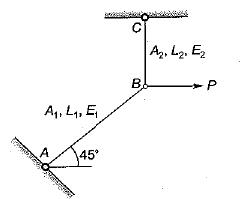Solution: